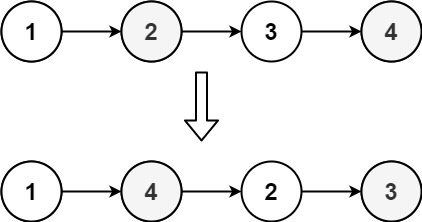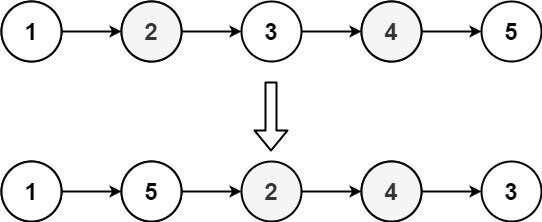## Algorithm

Problem Name: 143. Reorder List

You are given the head of a singly linked-list. The list can be represented as:

```L0 → L1 → … → Ln - 1 → Ln
```

Reorder the list to be on the following form:

```L0 → Ln → L1 → Ln - 1 → L2 → Ln - 2 → …
```

You may not modify the values in the list's nodes. Only nodes themselves may be changed.

Example 1:```Input: head = [1,2,3,4]
Output: [1,4,2,3]
```

Example 2:```Input: head = [1,2,3,4,5]
Output: [1,5,2,4,3]
```

Constraints:

• The number of nodes in the list is in the range `[1, 5 * 104]`.
• `1 <= Node.val <= 1000`

## Code Examples

### #1 Code Example with C Programming

```Code - C Programming```

``````
#include <stdio.h>
#include <stdlib.h>

struct ListNode {
int val;
struct ListNode *next;
};

struct ListNode *Helper(struct ListNode **forward, struct ListNode *node) {
if (node == NULL || node->next == NULL) return node;

struct ListNode *tail = Helper(forward, node->next);

if (*forward == tail || (*forward)->next == tail) return tail;

struct ListNode *t = (*forward)->next;
(*forward)->next = tail;
*forward = t;
tail->next = t;

return node;
}

struct ListNode *mid = Helper(&p, head);
mid->next = NULL;
}

struct ListNode *createNode(int new_data) {
struct ListNode *new_node = (struct ListNode *)malloc(sizeof(struct ListNode));
new_node->val = new_data;
new_node->next = NULL;
return new_node;
}

int main(){
/* test 1 */
struct ListNode *l1 = createNode(1);
struct ListNode *p = l1;
int i;
for (i = 2; i <= 5; i++) {
p->next = createNode(i);
p = p->next;
}
p->next = NULL;

printf("List  1: ");
p = l1;
while (p) {
printf("%d->", p->val);
p = p->next;
}
printf("NIL\n");

reorderList(l1);

printf("Reorder: ");
p = l1;
while (p) {
printf("%d->", p->val);
p = p->next;
}
printf("NIL\n");

/* test 2 */
struct ListNode *l2 = createNode(1);
p = l2;
for (i = 2; i <= 6; i++) {
p->next = createNode(i);
p = p->next;
}
p->next = NULL;

printf("List  2: ");
p = l2;
while (p) {
printf("%d->", p->val);
p = p->next;
}
printf("NIL\n");

reorderList(l2);

printf("Reorder: ");
p = l2;
while (p) {
printf("%d->", p->val);
p = p->next;
}
printf("NIL\n");

return 0;
}
``````
Copy The Code &

Input

cmd

Output

cmd
[1,4,2,3]

### #2 Code Example with Java Programming

```Code - Java Programming```

``````
class Solution {
while (fast != null && fast.next != null) {
slow = slow.next;
fast = fast.next.next;
}
ListNode secondHalf = reverse(slow.next);
slow.next = null;
while (firstHalf != null && secondHalf != null) {
ListNode firstHalfNext = firstHalf.next;
ListNode secondHalfNext = secondHalf.next;
firstHalf.next = secondHalf;
secondHalf.next = firstHalfNext;
firstHalf = firstHalfNext;
secondHalf = secondHalfNext;
}
}

private ListNode reverse(ListNode node) {
ListNode curr = node;
ListNode prev = null;
while (curr != null) {
ListNode next = curr.next;
curr.next = prev;
prev = curr;
curr = next;
}
return prev;
}
}
``````
Copy The Code &

Input

cmd

Output

cmd
[1,4,2,3]

### #3 Code Example with Javascript Programming

```Code - Javascript Programming```

``````
while(fast && fast.next) {
slow = slow.next
fast = fast.next.next
}
slow.next = null

}

function reverse(node) {
let pre = null, cur = node
while(cur) {
const tmp = cur.next
cur.next = pre
pre = cur
cur = tmp
}
return pre
}
};
``````
Copy The Code &

Input

cmd

Output

cmd
[1,5,2,4,3]

### #4 Code Example with Python Programming

```Code - Python Programming```

``````
class Solution(object):
arr = []
l, r, prev = 0, len(arr) - 1, ListNode(0)
while l < r: prev.next, arr[l].next, prev, l, r = arr[l], arr[r], arr[r], l + 1, r - 1
if l == r: prev.next = arr[l]
arr[l].next = None
``````
Copy The Code &

Input

cmd

Output

cmd
[1,5,2,4,3]

### #5 Code Example with C# Programming

```Code - C# Programming```

``````
namespace LeetCode
{
/**
* public class ListNode {
*     public int val;
*     public ListNode next;
*     public ListNode(int val=0, ListNode next=null) {
*         this.val = val;
*         this.next = next;
*     }
* }
*/
public class _0143_ReorderList
{
{

while (fast != null && fast.next != null)
{
slow = slow.next;
fast = fast.next.next;
}

ListNode prev = null, curr = slow, temp;
while (curr != null)
{
temp = curr.next;
curr.next = prev;
prev = curr;
curr = temp;
}

ListNode first = head, second = prev;
while (second.next != null)
{
temp = first.next;
first.next = second;
first = temp;

temp = second.next;
second.next = first;
second = temp;
}
}
}
}
``````
Copy The Code &

Input

cmd